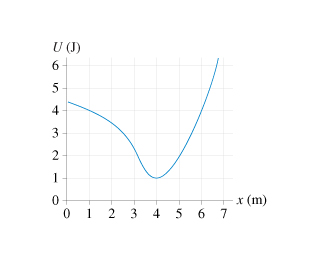# Problem:  is the potential-energy diagram for a 20 g particle that is released from rest at x = 1.0  m.a. What is the particles maximum speed? At what position does it have this speed?b. Where are the turning points of the motion?c. Will the particle move to the right or to the left?

###### FREE Expert Solution

Kinetic energy is found from the formula:

$\overline{){\mathbf{K}}{\mathbf{=}}\frac{\mathbf{1}}{\mathbf{2}}{{\mathbf{mv}}}^{{\mathbf{2}}}}$

a.

The maximum speed of the particle is found when the potential energy, U is minimum.

Potential energy, U is minimum at  x = 4m where U = 1 J

Applying the law of conservation of energy between points 4 and 1,

98% (145 ratings)###### Problem Detailsis the potential-energy diagram for a 20 g particle that is released from rest at x = 1.0  m.

a. What is the particles maximum speed? At what position does it have this speed?

b. Where are the turning points of the motion?

c. Will the particle move to the right or to the left?

Frequently Asked Questions

What scientific concept do you need to know in order to solve this problem?

Our tutors have indicated that to solve this problem you will need to apply the Force & Potential Energy concept. You can view video lessons to learn Force & Potential Energy. Or if you need more Force & Potential Energy practice, you can also practice Force & Potential Energy practice problems.

What professor is this problem relevant for?

Based on our data, we think this problem is relevant for Professor Schmitt's class at GWU.